## I, Oliver Bonham-Carter 👋

### Assistant Professor in Computer Science, Allegheny College

I, Oliver Bonham-Carter, In June

# T Tests

### Determining the difference between the means of two data sets.

How would you determine the difference between the averages following two datasets? We show a scatter plot and a histogram plot for each group (i.e., 1 and 2). Are these plots enough to determine a likeness between the plots?

But first, let’s get into a Jupyter client where we can run Python code.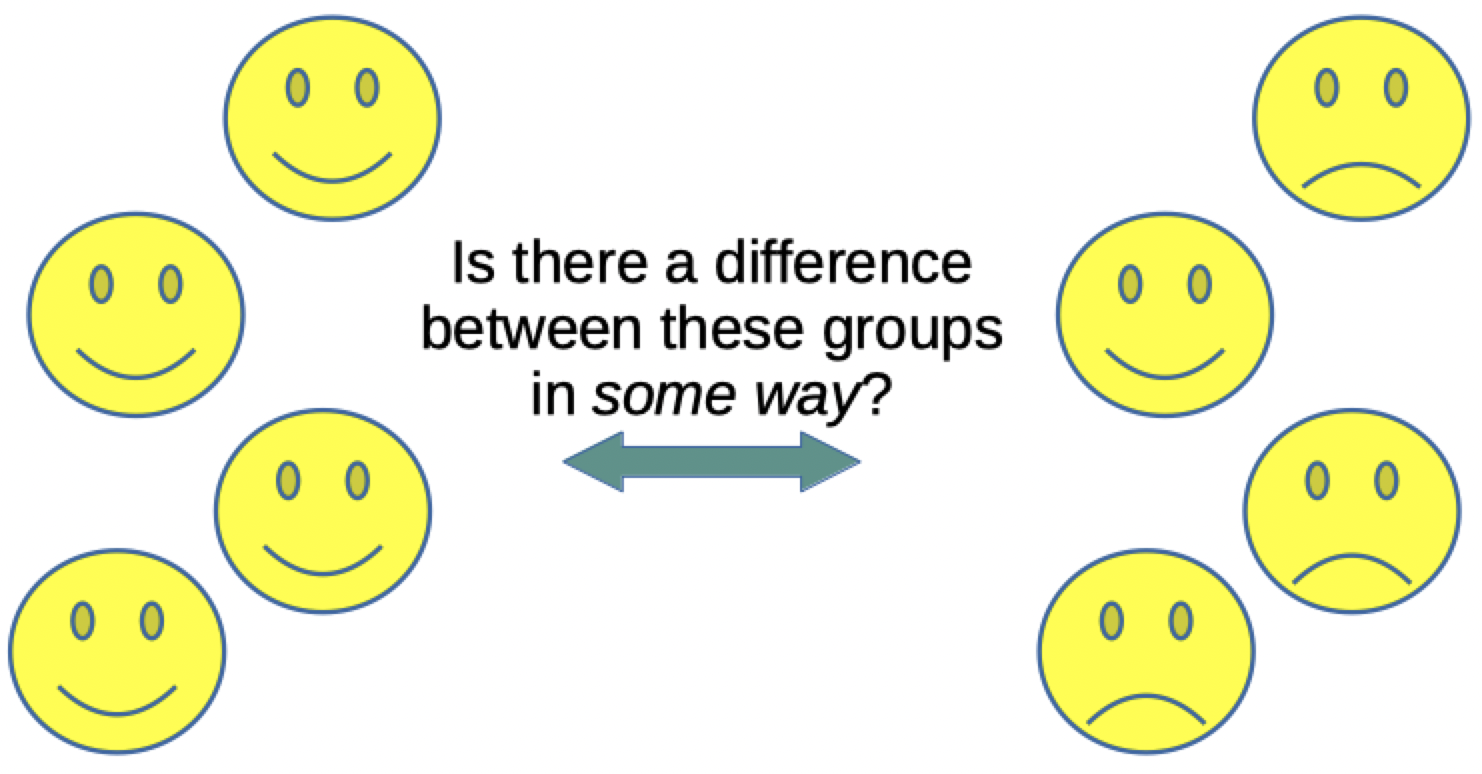### The Data

How could you differentiate the two data sets shown below? Could we simply average all numbers together and then use that value for a comparison? Yes, we could! The average would tell us that the two sets are not composed of the same values, but this singular value does not inform about the larger distribution qualities. For instance, can we study these numbers to ascertain a statistical difference between one set and the other?

#### Group 1 dataset

``````array([4.82311059, 5.64152115,
4.94118341, 4.51888519, 4.69452953,
4.99137589, 4.91842838, 6.37598021,
4.15584602, 6.55079577, 5.97303453,
6.95027014, 5.60942129, 4.92938286,
4.6932844, 5.26956469, 4.72835432,
4.71265135, 5.11285407, 6.93112916,
2.6664159 , 5.96984681, 5.61169188,
2.92437265, 6.25106099, 5.46805324,
5.38712146, 4.56640858, 5.18326553,
5.65786142, 4.69512564, 3.86439402,
7.37302804, 6.1169038 , 4.38961873,
6.27314289, 5.88551304, 5.34850487,
5.13206916, 7.61189171, 5.17720053,
5.88717038, 3.62097149, 3.02204612,
4.92681691, 4.31055542, 4.51201151,
4.2912872 , 4.63346511, 5.42599103])
``````

#### Group 2 dataset

``````array([ 9.34838992,  7.59303945,
7.32399982, 6.19395197, 6.75827388,
8.22905613, 8.90642506, 7.19739203,
6.44502027, 8.73127784, 5.99917675,
8.79211838, 6.93085735, 7.53288447,
7.86203899, 7.97850888, 8.05638105,
8.52991009, 7.7587821,  9.17111222,
6.34387003, 8.32597246, 8.36348416,
8.32186526, 8.5008081 , 9.15884336,
9.3251948 , 7.52155757, 8.51486734,
6.02272479, 8.20096257, 6.58747512,
7.45891872, 8.22118695, 9.70966141,
8.18894409, 8.60399128, 7.0688741 ,
5.72850754, 7.30309111, 10.25824742,
8.48691697, 7.91207794, 8.72347449,
7.99540331, 8.51854714, 8.97678889,
7.7033359, 7.72142998, 9.0834914 ])
``````

### What do the plots look like?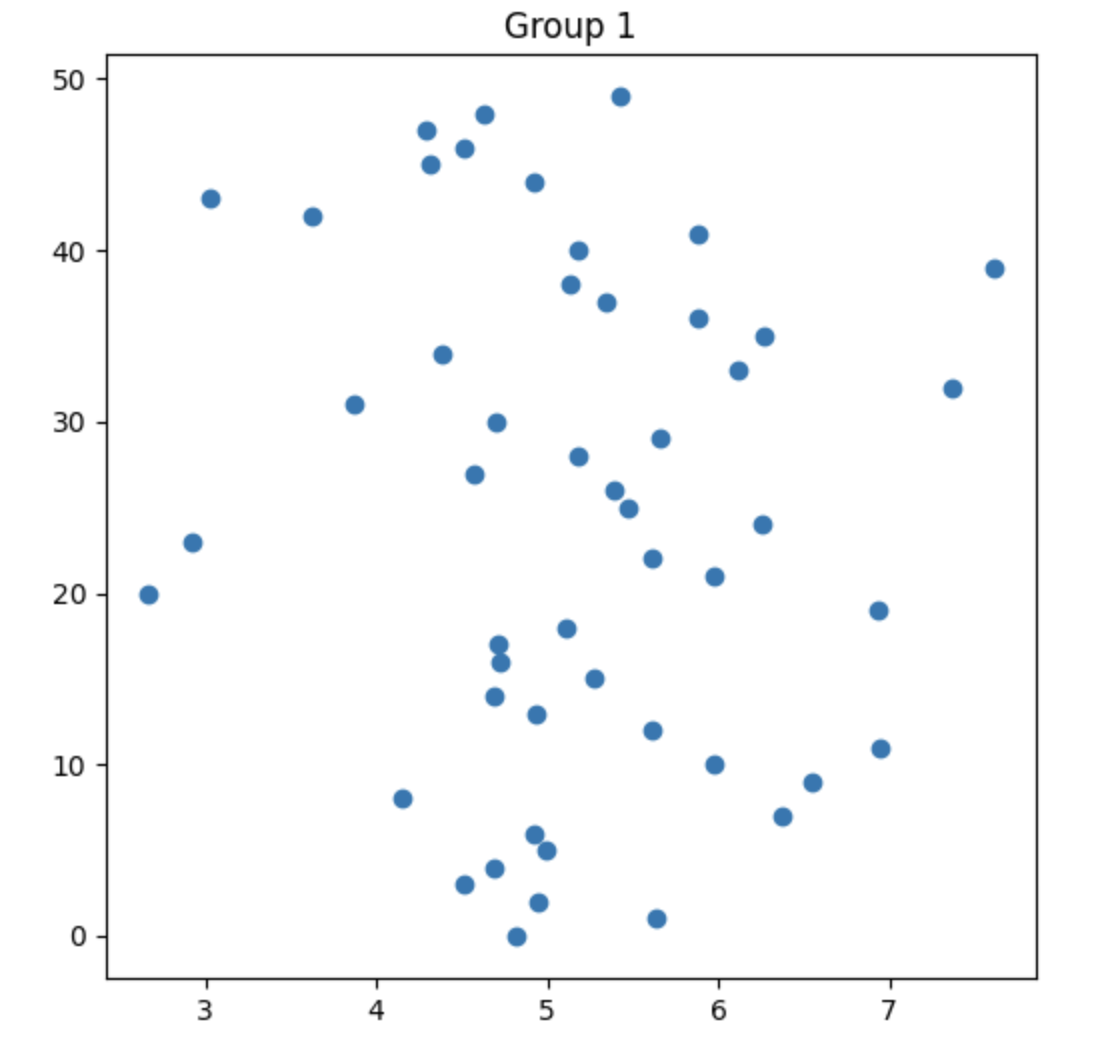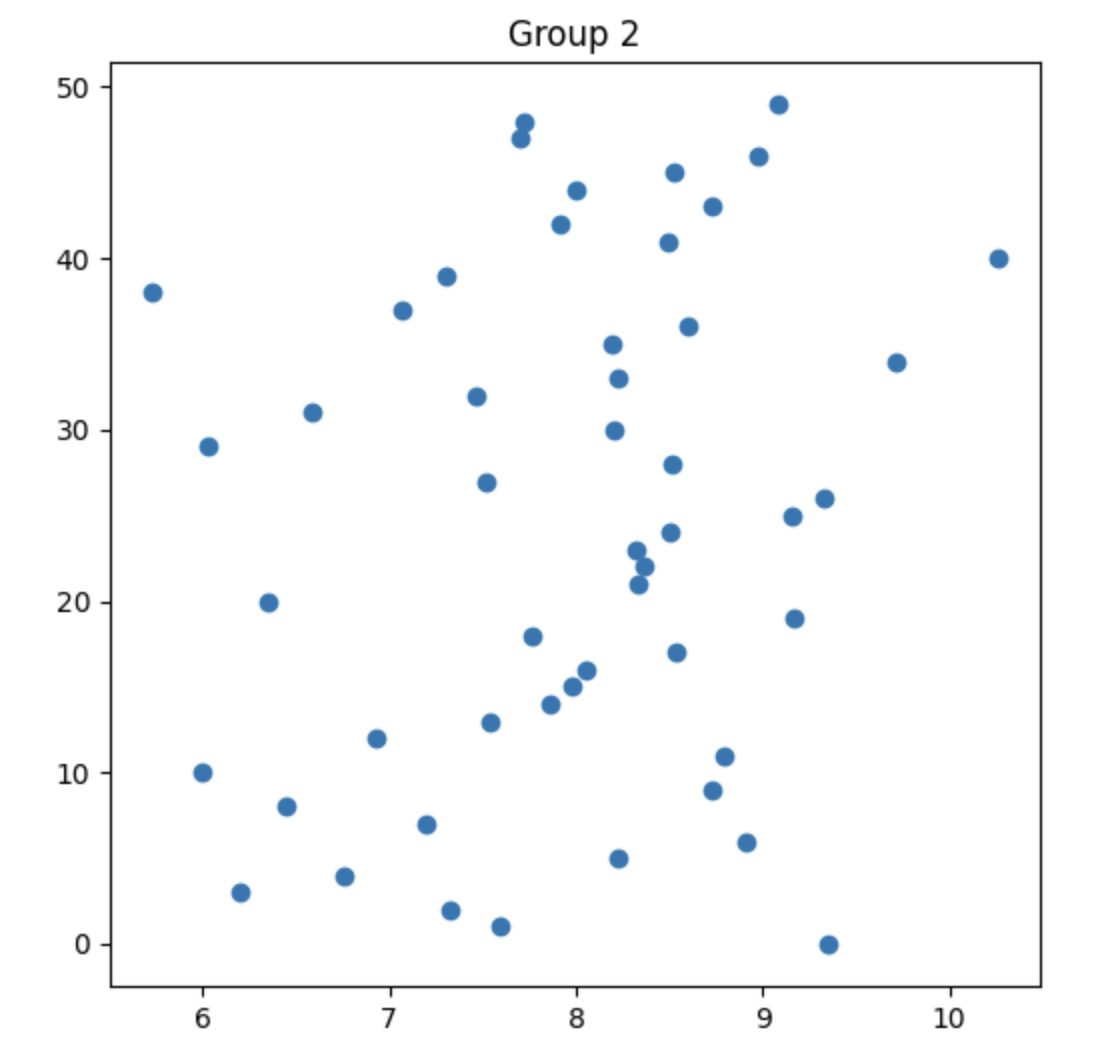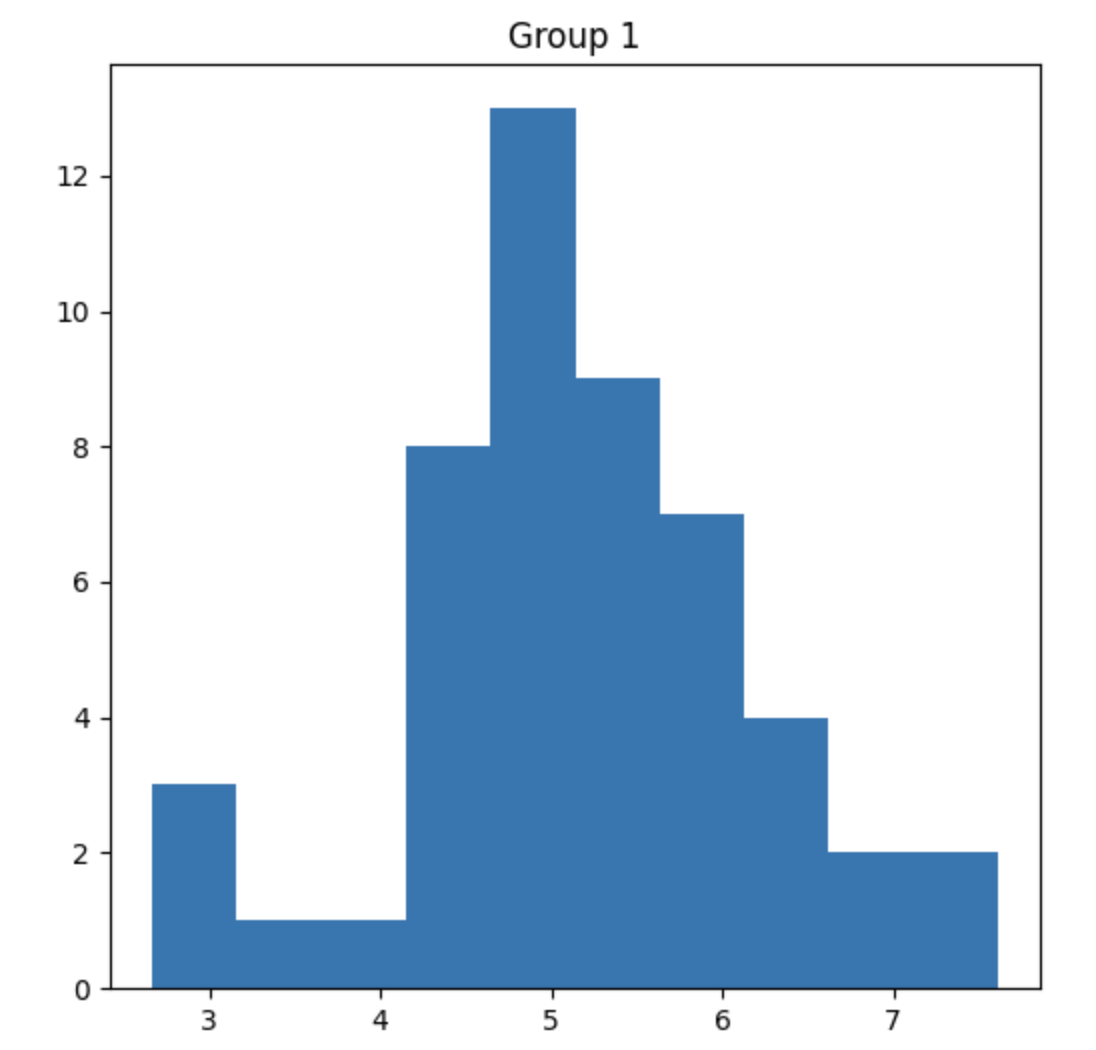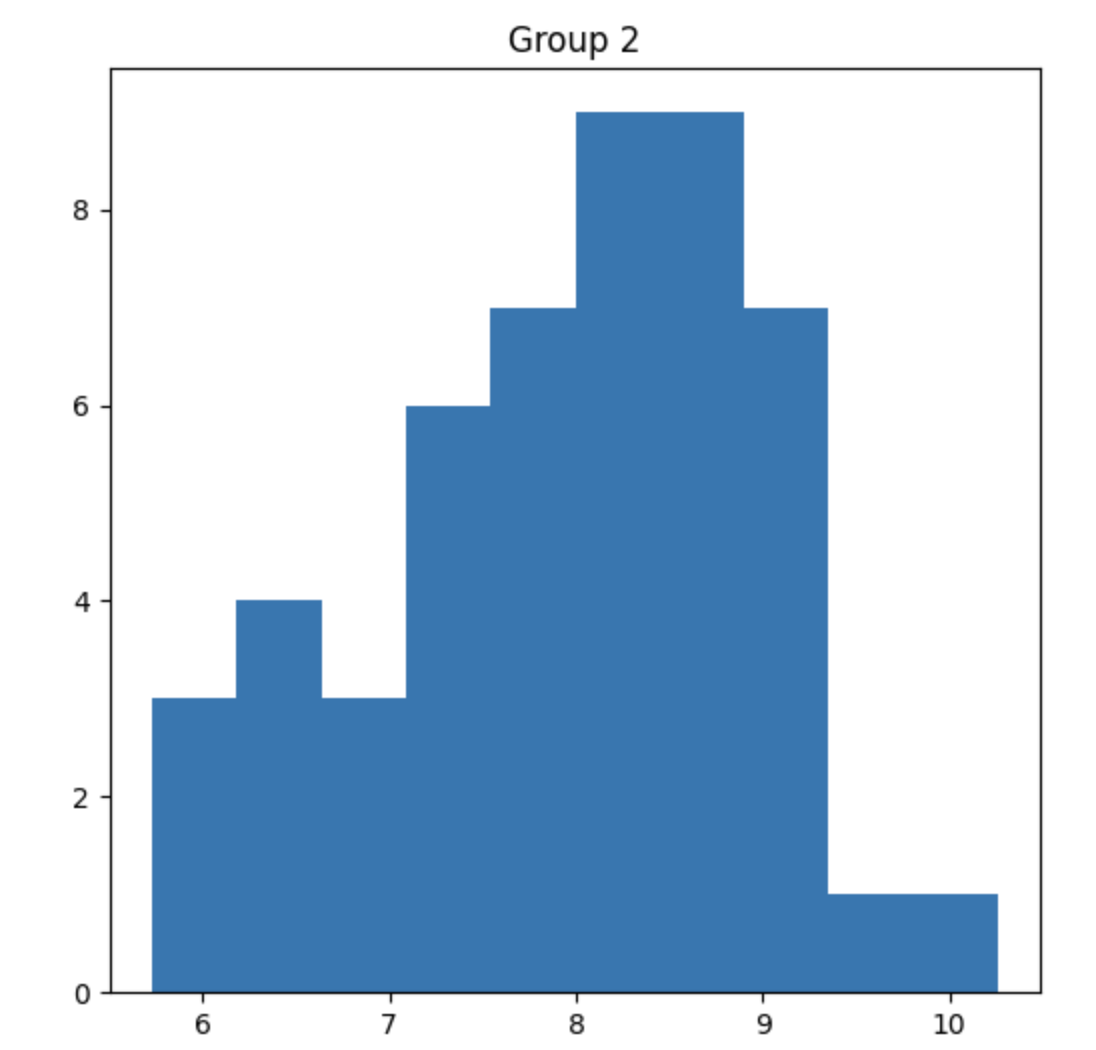### What are we actually asking? What are our Hypotheses?

Is there a statistically significant difference between the two groups in terms of the average extent to which the bottles are filled?

• Null hypothesis (Ho):

• The datasets have the same range of values. (Nothing unusual is happening.)
• Alternative hypothesis (Ha):

• There is a difference between the ranges of values in the datasets.

### Which hypothesis do we accept?!

We complete a statistical test to tell us which hypothesis to accept. We use a value from the output of the test called the p-value to inform us which is the most likely hypothesis to accept. Below, we see a number line which shows us that when the p-value is really small, then we accept the Alternative hypothesis. Otherwise, we take the Null hypothesis.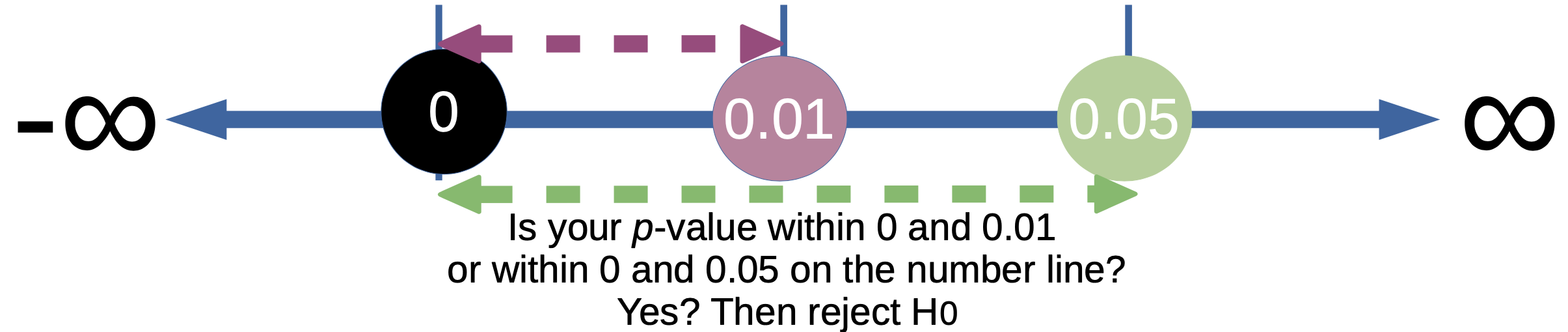When the p-value is between 0 and 0.01, or when the p-value is between 0 and 0.05, then we reject the null hypothesis (and take the alternative hypothesis.) Otherwise, we accept the Null hypothesis.

### Description of code

In this example, we first generate two sets of sample data using NumPy’s random.normal function. We then use the t-test_ind function from the SciPy library to calculate the t-statistic and p-value. Finally, we output the results, which include the means of the two groups, the t-statistic, and the p-value.

Note that the t-test_ind function assumes that the two groups have equal variances. If this is not the case, you may need to use a different t-test function or perform some additional steps to correct for unequal variances.

``````import numpy as np
from scipy.stats import ttest_ind

# Generate two sets of sample data
group1 = np.random.normal(5, 1, size=50)
group2 = np.random.normal(7, 1, size=50)

# Calculate the t-statistic
# and p-value using ttest_ind from SciPy

t_statistic, p_value = ttest_ind(group1, group2)

# Output the results
print("Group 1 mean:", np.mean(group1))
print("Group 2 mean:", np.mean(group2))
print("T-Statistic:", t_statistic)
print("P-Value:", p_value)
``````

Read the p-value to see what to do!

### Same t-test With Histogram Plots

``````import numpy as np
from scipy.stats import ttest_ind
import matplotlib.pyplot as plt

# Generate two sets of sample data
group1 = np.random.normal(5, 1, size=50)
group2 = np.random.normal(7, 1, size=50)

plt.hist(group1)
plt.show()

plt.hist(group2)
plt.show()

# Calculate the t-statistic
# and p-value using ttest_ind from SciPy

t_statistic, p_value = ttest_ind(group1, group2)

# Output the results
print("Group 1 mean:", np.mean(group1))
print("Group 2 mean:", np.mean(group2))
print("T-Statistic:", t_statistic)
print("P-Value:", p_value)
``````

Read the p-value to see what to do!

### Same t-test With Histogram and Scatter Plots

``````import numpy as np
from scipy.stats import ttest_ind

import matplotlib.pyplot as plt

def getMyYAxis(in_list):
""" function to find a y-value for each x-value """
return [i for i in range(len(in_list))]
# end of getmyY

# Generate two sets of sample data

# same types of data sets
# Generate two sets of sample data
# group1 = np.random.normal(5, 1, size=50)
# group2 = np.random.normal(5, 1, size=50)

# Different types of data sets
# Generate two sets of sample data
group1 = np.random.normal(5, 1, size=50)
group2 = np.random.normal(8, 1, size=50)

myX = group1
myY =  getMyYAxis(group2)
plt.title('Group 1')
plt.scatter(x = myX, y = myY)
plt.show()

myX = group2
plt.title('Group 2')
myY =  getMyYAxis(group2)
plt.scatter(x = myX, y = myY)
plt.show()

plt.title('Group 1')
plt.hist(group1)
plt.show()

plt.title('Group 2')
plt.hist(group2)
plt.show()

# Calculate the t-statistic
# and p-value using ttest_ind from SciPy

t_statistic, p_value = ttest_ind(group1, group2)

# Output the results
print("Group 1 mean:", np.mean(group1))
print("Group 2 mean:", np.mean(group2))
print("T-Statistic:", t_statistic)
print("P-Value:", p_value)
``````

Read the p-value to see what to do!

### Conclusions

By using the p-value after running a t-test, we can determine which hypothesis to accept with some confidence. If you are interested in learning more about t-tests (the one and two tailed tests, for instance), then please check out the below links!